# How to Create Packages in Oracle

## How to Create Packages in Oracle with Examples?

In this article, I am going to discuss How to Create Packages in Oracle with Examples. Please read our previous article where we discussed the basic concepts of Packages in Oracle.

##### How to Create Packages in Oracle?

We will take the real example of using packages. Let’s create a table called student. We will create the columns like student_id, first_name, birthday, and a constraint primary key which is student_id.

```create table student
(student_id number,
first_name varchar2(100),
birthday date,
constraint student_pk primary key (student_id)
);
```

Let’s go ahead and create this table.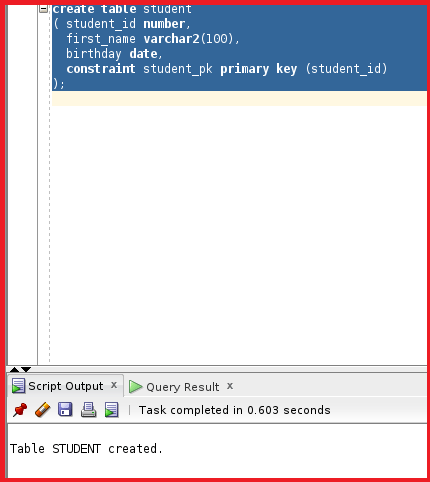So, the table STUDENT is created. Let’s create a sequence and will use this sequence for insert purposes.

create sequence student_seq;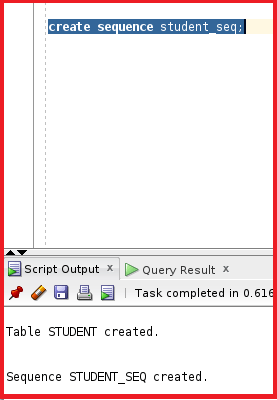So, the sequence is created. Now, let’s create a package for inserting, deleting, and querying a student. So, the package contains three subprograms.

```create or replace package General_student
is
procedure insert_student
(p_first_name varchar2, p_birthday date);

procedure delete_student
(p_student_id number);

function  get_name
(p_student_id number )
return varchar2;
end;
```

From the above package specification, we can see the package name is General_Student where we have three procedure headers. We have a procedure for insert_student with parameters p_first_name with varchar2 and p_birthday with the date as the datatypes. The second procedure is delete_student which takes p_student_id as the parameter where the datatype is a number. The third sub-program is the function that takes the p_student_id as the input and returns the name as the output. Let’s compile the package.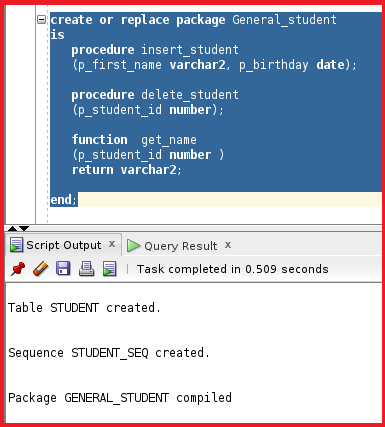So, the package is created. Let’s go ahead and create the package body.

```create or replace package body General_student
is
procedure insert_student
(p_first_name varchar2, p_birthday date)
is
begin
insert into student
values (student_seq.nextval,p_first_name,p_birthday);
commit;

exception
when others then
dbms_output.put_line(sqlcode);
dbms_output.put_line(sqlerrm);
end;

procedure delete_student
(p_student_id number)
is
begin
delete from student
where student_id =p_student_id ;
commit;

exception
when others then
dbms_output.put_line(sqlcode);
dbms_output.put_line(sqlerrm);
end;

function  get_name
(p_student_id number )
return varchar2
is
v_name student.first_name%type;
begin
select first_name into v_name
from student
where student_id=p_student_id;

return v_name;

exception
when others then
return null;
end;
end;
```

We have three sub-programs with two procedures and one function. Let’s go ahead and see each sub-program separately.

##### Procedure 1:
```procedure insert_student
(p_first_name varchar2, p_birthday date)
is
begin
insert into student
values (student_seq.nextval,p_first_name,p_birthday);
commit;

exception
when others then
dbms_output.put_line(sqlcode);
dbms_output.put_line(sqlerrm);
end;
```

We have the procedure insert_student which takes two parameters p_first_name and p_birthday and inserts them into the table student where the values are the sequence which is considered as the student_id and the first_name and the birthday. We do have an exception that displays if there are any errors.

##### Procedure 2:
```procedure delete_student
(p_student_id number)
is
begin
delete from student
where student_id =p_student_id ;
commit;

exception
when others then
dbms_output.put_line(sqlcode);
dbms_output.put_line(sqlerrm);
end;
```

The procedure delete_student which takes p_student_id as the input and deletes the records from the table student. This procedure matches the student_id with p_student_id and then deletes the record in the student table. There is an exception column inside the exception.

##### Function:
```function  get_name
(p_student_id number )
return varchar2
is
v_name student.first_name%type;
begin
select first_name into v_name
from student
where student_id=p_student_id;

return v_name;

exception
when others then
return null;
end;
```

The function takes student_id as the input and then selects the first_name from the student table where the student_id=p_student_id and then returns the student_name. There is an exception if there is no data print null. This is the entire package body. Let’s go ahead and compile this package body.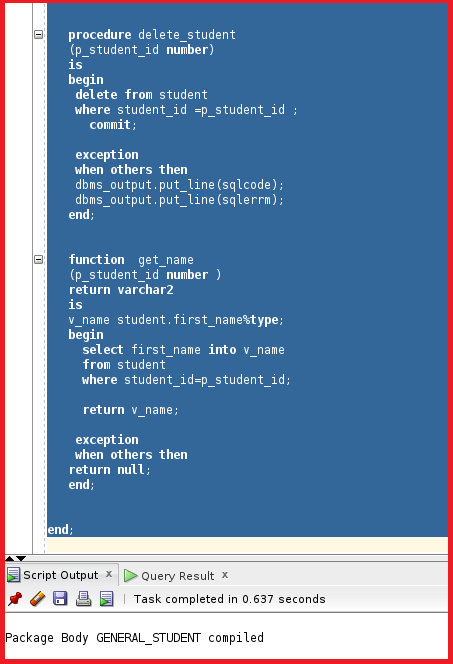So, the package body is now compiled. So, now let’s test this package.

execute general_student.insert_student (‘Jay’, ’20-Jul-97′);

We are using the insert_student procedure which takes two input parameters. The input takes first_name and birthday.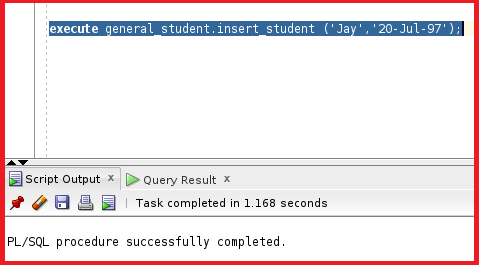So, the insert_student inside the package is executed successfully. This indicates the records are inserted into the table student. Let’s go ahead and add another record to the table.

execute general_student.insert_student (‘Ram’, ’10-Aug-96′);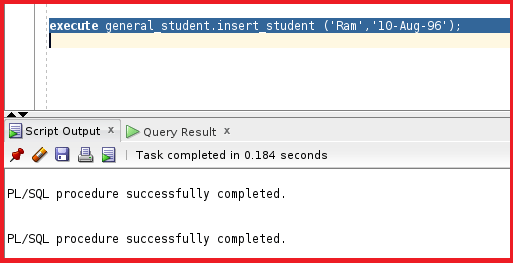So, the other record is also inserted successfully. Let’s now check the table students.

select * from student;

So, we can see two records that are present inside the table student. Let’s now check the delete_student procedure.

execute general_student.delete_statement(1);

So, the delete_student procedure is executed successfully. We have given the input parameter as 1 which checks for the student_id as 1 and deletes the record with student_id as 1. Let’s now check the table students to see if the record is deleted or not.

select * from student;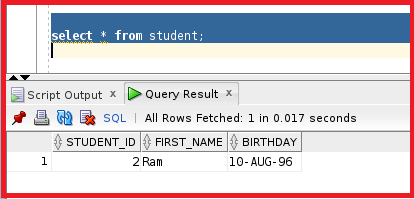So, the record with student_id 1 is deleted. So, the delete_Student procedure is also working fine. Let’s test the function inside the package.

select general_student.get_name(2) from dual;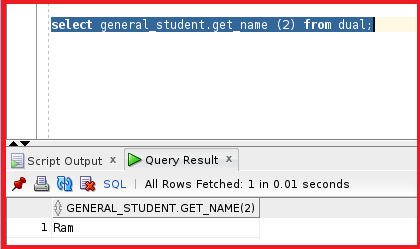So, we can see the details of the student Ram. The function is also working fine. So, we have learned how to create a package where we include multiple sub-programs inside the package.

In the next article, I am going to discuss How to Create Package Specifications without a Body in Oracle with Examples. Here, in this article, I try to explain How to Create Packages in Oracle with Examples. I hope you enjoy this How to Create Packages in Oracle with Examples article.